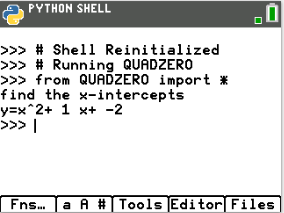# Activities

•• ##### Subject Area

• Math: Explorations with Coding: Coding with Python

6-8
9-12

• ##### Device
•TI-84 Plus CE Python
• ##### Report an Issue#### Activity Overview

In this coding activity for the TI-84 Plus CE Python edition, students will explore factored equations, and create a game to practice finding integer x-intercepts for equations.

#### Objectives

• Use the input function and a variable to collect and store data from a user
• Use the randint() function to generate random integers.
• Use a while loop to repeat code
• Use if..elif..else statements to make decisions.
• Explore how x-intercepts are related to factored quadratic equations
• Explore how to factor equation in standard form
• Factor quadratic equations with integer solutions